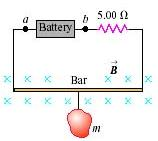# Problem: The circuit shown in the drawing is used to make a magnetic balance to weigh objects. The mass m to be measured is hung from the center of a bar, which is in a uniform magnetic field of 1.50 T directed into the page. The battery voltage can be adjusted to vary the current in the circuit. The horizontal bar is 60.0 cm long and is made of extremely lightweight material, so its mass can be neglected. It is connected to the battery by thin vertical wires that can support no appreciable tension; that is, all the weight of the mass m is supported by the magnetic force on the bar. A 5.00 Ω resistor is in series with the bar, and the resistance of the rest of the circuit is negligibly small. (a) Which point, a or b, should be the positive terminal of the battery? (b) If the maximum terminal voltage of the battery is 175 V, what is the greatest mass m that this instrument can measure?

###### FREE Expert Solution

(a) The direction of magnetic force:

$\overline{)\stackrel{\mathbf{⇀}}{\mathbf{F}}{\mathbf{=}}{\mathbf{I}}{\mathbf{\left(}}\stackrel{\mathbf{⇀}}{\mathbf{L}}{\mathbf{×}}\stackrel{\mathbf{⇀}}{\mathbf{B}}{\mathbf{\right)}}}$

From right-hand rule, the current is in an anticlockwise direction.

92% (245 ratings)###### Problem DetailsThe circuit shown in the drawing is used to make a magnetic balance to weigh objects. The mass m to be measured is hung from the center of a bar, which is in a uniform magnetic field of 1.50 T directed into the page. The battery voltage can be adjusted to vary the current in the circuit. The horizontal bar is 60.0 cm long and is made of extremely lightweight material, so its mass can be neglected. It is connected to the battery by thin vertical wires that can support no appreciable tension; that is, all the weight of the mass m is supported by the magnetic force on the bar. A 5.00 Ω resistor is in series with the bar, and the resistance of the rest of the circuit is negligibly small.

(a) Which point, a or b, should be the positive terminal of the battery?

(b) If the maximum terminal voltage of the battery is 175 V, what is the greatest mass m that this instrument can measure?